Home Practice
For learners and parents For teachers and schools
Textbooks
Full catalogue
Pricing SupportLog in

We think you are located in United States. Is this correct?

# 8.6 Pythagorean theorem

## 8.6 Pythagorean theorem (EMCJH)

temp text

Many different methods of proving the theorem of Pythagoras have been formulated over the years. Similarity of triangles is one method that provides a neat proof of this important theorem.

# Chapter 8: Theorem: Pythagorean theorem

The square on the hypotenuse of a right-angled triangle is equal to the sum of the squares on the other two sides.

(Reason: Pythagoras or $$\text{right-angled } \triangle \text{s}$$)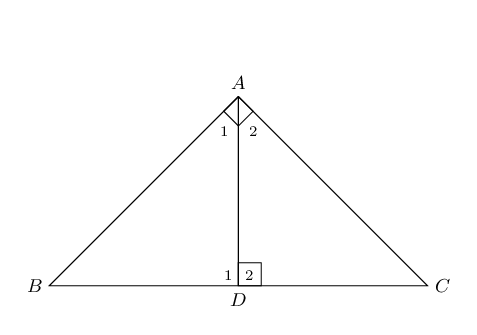$$\triangle ABC$$ with $$\hat{A}=\text{90}°$$

$$B{C}^{2}=A{B}^{2}+A{C}^{2}$$

Construction: draw $$AD \perp BC$$.

$\begin{array}{rll} \hat{C} + \hat{A}_{2} & = \text{90}° & \text{(}\angle \text{s}\text{ of }\triangle CAD \text{)} \\ \hat{A}_{1} + \hat{A}_{2} & = \text{90}° & \text{(given)} \\ \therefore \hat{A}_{1} & = \hat{C} & \\ & & \\ \hat{A}_{1} + \hat{B} & = \text{90}° & \text{(}\angle \text{s}\text{ of }\triangle ABD \text{)} \\ \therefore \hat{B} & = \hat{A}_{2} & \\ & & \\ \hat{D}_{1} & = \hat{D}_{2}= \hat{A} = \text{90}° & \text{(construction)} \\ & & \\ \therefore \triangle ABD \enspace & ||| \enspace \triangle CBA \enspace ||| \enspace \triangle CAD &\text{(AAA)} \\ & & \\ \therefore \frac{AB}{BC}&=\frac{BD}{AB} & (\triangle ABD \enspace ||| \enspace \triangle CBA ) \\ & & \\ AB^{2} &= BD \times BC & \\ & & \\ \text{Similarly } \frac{AC}{CB}&=\frac{DC}{AC} & (\triangle ABD \enspace ||| \enspace \triangle CBA ) \\ & & \\ AC^{2} &= CB \times DC &\\ & & \\ \therefore AC^{2} + AB^{2} &= (BD \times BC) + (CB \times DC ) &\\ &= BC(BD + DC ) & \\ &= BC(BC ) & \\ &= BC^{2}& \\ & & \\ \therefore BC^{2} &= AC^{2} + AB^{2} & \\ \end{array}$

Converse: theorem of Pythagoras

If the square of one side of a triangle is equal to the sum of the squares of the other two sides of the triangle, then the angle included by these two sides is a right angle.

## Worked example 8: Theorem of Pythagoras

In $$\triangle PQR$$, $$P\hat{Q}R = \text{90}°$$ and $$QT \perp PR$$. If $$PQ = \text{7}\text{ cm}$$ and $$QT = 3\sqrt{5} \enspace \text{cm}$$, determine $$PR$$ and $$QR$$ (correct to the nearest integer).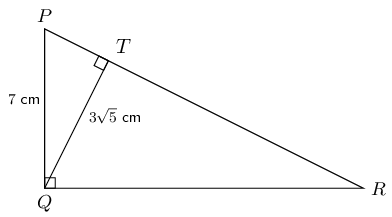### Use the theorem of Pythagoras to determine $$PT$$

$\begin{array}{rll} \text{In } \triangle PTQ, \quad PT^{2} &= PQ^{2} - QT^{2} & (\text{Pythagoras}) \\ &= 7^{2} - \left( 3\sqrt{5} \right)^{2} & \\ &= 49 - 45 & \\ \therefore PT &= \sqrt{4} & \\ &= \text{2}\text{ cm} & \end{array}$

### Use proportionality to determine $$PR$$ and $$QR$$

$\begin{array}{rll} P\hat{Q}R &=\text{90}° & \text{(given)} \\ QT &\perp PR & \text{(given)} \\ \therefore \enspace \triangle PQT \enspace & ||| \enspace \triangle QRT \enspace ||| \enspace \triangle PRQ & (\text{right-angled } \triangle \text{s}) \\ & & \\ \therefore \dfrac{QT}{TR} &= \dfrac{PT}{QT} & (\triangle QRT \enspace ||| \enspace \triangle PQT ) \\ & & \\ \therefore QT^{2} &= TR \cdot PT & \\ & & \\ \left( 3\sqrt{5} \right)^{2} &= TR \cdot 2 & \\ & & \\ \frac{45}{2} &= TR & \\ & & \\ \text{And } PR &= PT + TR & \\ &= \dfrac{45}{2} + 2 & \\ &= \text{25}\text{ cm} & \text{(to nearest integer)}\\ & & \\ \text{In } \triangle PQR, \quad QR^{2} &= PR^{2} - PQ^{2} & (\text{Pythagoras}) \\ &= 25^{2} - 7^{2} & \\ \therefore QR &= \sqrt{576} & \\ &= \text{24}\text{ cm} & \end{array}$

$$PR = \text{25}\text{ cm}$$ and $$QR = \text{24}\text{ cm}$$

For any right-angled $$\triangle MNP$$, if $$MQ$$ is drawn perpendicular to $$NP$$, then: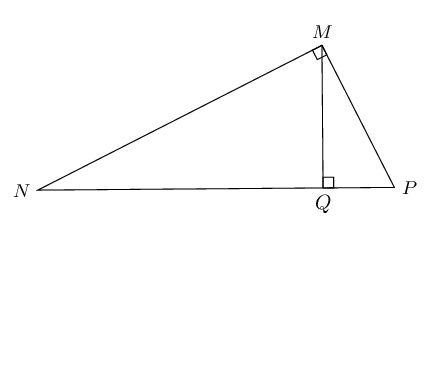## Theorem of Pythagoras

Textbook Exercise 8.9

$$B$$ is a point on circle with centre $$O$$. $$BD \perp AC$$ and $$D$$ is the midpoint of radius $$OC$$.

If the diameter of the circle is $$\text{24}\text{ cm}$$, find $$BD$$.

Leave answer in simplified surd form.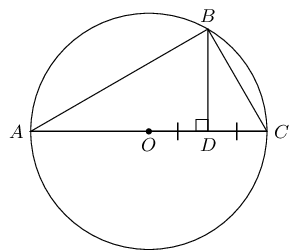$\begin{array}{rll} ABC&= \text{90}° & (\angle \text{ in semi-circle}) \\ BD&\perp AC & \\ \therefore BD^{2}&= AC.DC & \\ AO &= OC & (\text{equal radii}) \\ &= \frac{1}{2} AC & \\ &= \text{12}\text{ cm} & \\ \therefore DC &= \text{6}\text{ cm} & \\ \therefore AD&= \text{18}\text{ cm} & \\ BD^{2}&= AD \cdot DC & (\text{right-angled } \triangle \text{s}) \\ BD^{2}&= 18 \times 6 & \\ BD&= \sqrt{108} & \\ &= \sqrt{36 \times 3} & \\ &= 6 \sqrt{3} \text{ cm} & \end{array}$

In $$\triangle PQR$$, $$RQ \perp QP$$ and $$QT \perp RP$$. $$PQ = 2$$ units, $$QR = b$$ units, $$RT = 3$$ units and $$TP = a$$ units. Determine $$a$$ and $$b$$, giving reasons.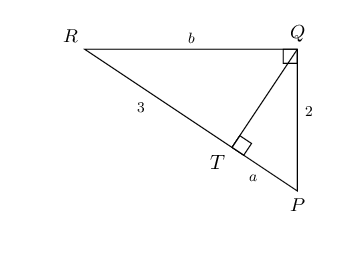$\begin{array}{rll} QP^2 &= PT \cdot PR & (\text{right-angled } \triangle \text{s}) \\ 2^{2} &= a(a + 3) & \\ 4 &= a^{2} + 3a & \\ 0 &= a^{2} + 3a -4 & \\ 0 &= (a - 1)(a + 4) & \\ \therefore a &= 1 \text{ or } a = -4 & \\ \text{Length must be positive } & \therefore a = 1 \text{ unit} & \end{array}$ $\begin{array}{rll} QR^2 &= RT \cdot RP & (\text{right-angled } \triangle \text{s}) \\ b^{2} &= 3(3 + 1) & \\ &= 3(4) & \\ &= 12 & \\ \therefore b &= \pm \sqrt{12} & \\ \text{Length must be positive } & \therefore b = 2\sqrt{3} \text{ units} & \end{array}$

Chord $$AQ$$ of circle with centre $$O$$ cuts $$BC$$ at right angles at point $$P$$.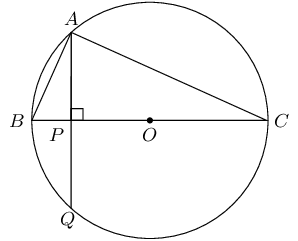Why is $$\triangle ABP \enspace ||| \enspace \triangle CBA$$?

$\begin{array}{rll} B\hat{A}C&= \text{90}° & (BC \text{ is diameter of circle }O) \\ \end{array}$

In $$\triangle ABP$$ and $$\triangle CBA$$:

$\begin{array}{rll} B\hat{P}A&= B\hat{A}C =\text{90}° & (\text{given}) \\ \hat{B}&= \hat{B} & (\text{common } \angle) \\ \therefore \triangle ABP& \enspace ||| \enspace \triangle CBA & (\text{AAA}) \end{array}$

If $$AB = \sqrt{6}$$ units and $$PO = 2$$ units, calculate the radius of the circle.

In $$\triangle ABP$$:

$\begin{array}{rll} AP^{2} &= BA^{2} - BP^{2} & (\text{Pythagoras}) \\ BP &= BO - PO & \\ &= r - 2 & (BO = r, \text{ given } PO = 2) \\ AP^{2} &= \left( \sqrt{6} \right)^{2} - (r-2)^{2} & \\ &= 6 - (r-2)^{2} & \\ & & \\ AP^{2} &= BP.PC & (B\hat{A}C = \text{90}°, AP \perp BC) \\ &= (r-2).PC & \\ \text{And } PC &= PO + OC & \\ &= 2 + r & \\ \therefore AP^{2} &= (r-2)(2 + r) & \end{array}$ $\begin{array}{rll} 6 - (r-2)^{2} &= (r-2)(2 + r) & \\ 6 - r^{2} + 4r - 4 &= r^{2} - 4 & \\ 2r^{2} - 4r -6 &= 0 & \\ r^{2} - 2r - 3 &= 0 & \\ (r-3)(r+1) &= 0 & \\ r = 3 \text{ or } r &= -1 & \\ \therefore r &= \text{3}\text{ units} & \\ \end{array}$

In the diagram below, $$XZ$$ and $$WZ$$ are tangents to the circle with centre $$O$$ and $$X\hat{Y}Z = \text{90}°$$.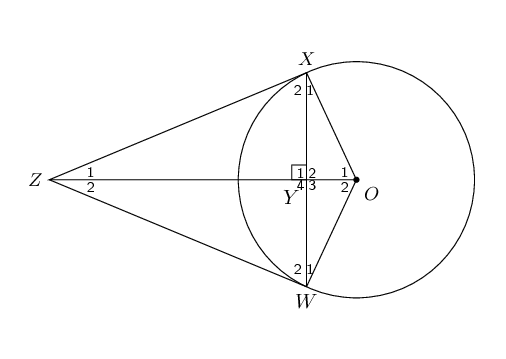Show that $$XY^{2} = OY \cdot YZ$$.

$\begin{array}{rll} \text{In } \triangle OXZ: & & \\ X\hat{Y}Z & = \text{90}° & (\text{given}) \\ O\hat{X}Z & = \text{90}° & (\text{tangent } \perp \text{ radius}) \\ \therefore XY^{2} & = OY \cdot YZ & (\text{right-angled } \triangle \text{s}) \end{array}$

Prove that $$\frac{OY}{YZ} = \frac{OW^{2}}{WZ^{2}}$$.

$\begin{array}{rll} \text{In } \triangle OWZ: & & \\ W\hat{Y}Z & = \text{90}° & (\text{given}) \\ O\hat{W}Z & = \text{90}° & (\text{tangent } \perp \text{ radius}) \\ \therefore WZ^{2} & = ZY \cdot ZO & (\text{right-angled } \triangle \text{s}) \\ \text{And } WO^{2} & = OY \cdot ZO & (\text{right-angled } \triangle \text{s}) \\ \therefore \frac{WO^{2}}{WZ^{2}} & = \frac{OY \cdot ZO}{ZY \cdot ZO} & \\ \frac{WO^{2}}{WZ^{2}} & = \frac{OY}{ZY} & \\ \therefore \frac{OY}{YZ} &= \frac{OW^{2}}{WZ^{2}} & \end{array}$